Starts With A Bang

# Ask Ethan: Could Octonions Unlock How Reality Really Works?

#### A fascinating mathematical structure exists that goes well beyond our common experience. Could it revolutionize physics?

Perhaps the most remarkable fact about the Universe is that every particle within it — at all times, locations, and under all conditions — obeys the exact same laws of physics. The rules that nature plays by are the same for everyone, and by finding the mathematical structure that describes those rules, we can describe nature as well. Oftentimes, discovering a new mathematical structure leads to the development of a new physical framework, and where that framework accurately describes the Universe, new physics can be deduced. One of the most fascinating mathematical possibilities for our Universe involves something known as octonions, and that brings us to Patreon supporter Pedro Teixeira’s question, which is:

Octonions, do they stand a chance of being the answer to how our reality works, or just hype?

Let’s start at the beginning: with the mathematics that underlies physics.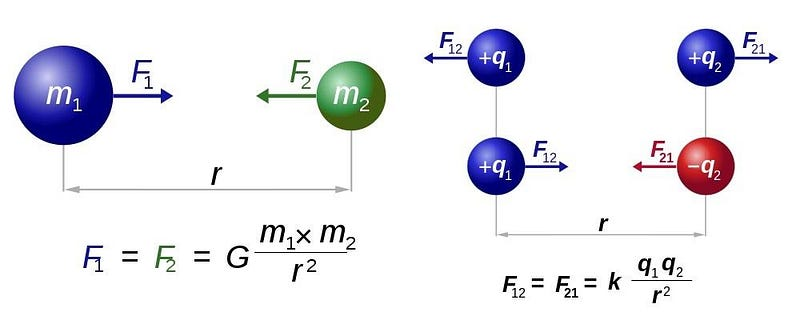Newton’s law of universal gravitation (L) and Coulomb’s law for electrostatics (R) have almost identical forms to their force laws, which can be solved to yield equations of motion for particles in the classical picture of the Universe. No mathematics more advanced than real numbers are needed to solve these equations. (DENNIS NILSSON / RJB1 / E. SIEGEL)

If all you had at your mathematical disposal was the idea of real numbers, you could still get very far. From Galileo to Newton to Coulomb to Maxwell, the entirety of classical physics is built on the basis of real numbers. Force laws, equations of motion, and much more can be derived without resorting to mathematics any more advanced than the set of real numbers, including variables, constants, and dependent functions.

But this already requires a mathematical leap that took millennia to develop: the leap to include negative numbers. When you throw a ball in the air and ask when it will hit the ground, you get two answers for time: one positive and one negative. Sometimes, either answer can be correct, but mathematics alone won’t tell you which situation applies. For that, you need the physical conditions of the problem, and that’s how you decide which answer is the relevant one.By examining this strobe image of a bouncing ball, you cannot tell for certain whether the ball is moving towards the right and losing energy with each bounce, or whether it’s moving towards the left and getting an energetic kick with each bounce. The laws of physics are symmetric under time-reversal transformations, and the equations of motion will give you two solutions (positive and negative) to any trajectory you can derive. Only by imposing physical constraints can we know which of the two yields the correct answer. (WIKIMEDIA COMMONS USERS MICHAELMAGGS AND (EDITED BY) RICHARD BARTZ)

However, real numbers ⁠ — even when you include both positive and negative numbers ⁠ — have a limit to the complexity of their mathematical structure. For example, any real number, when you square it, always gives you a positive number, regardless of whether the real number you started with was positive or negative. If you attempt to take a square root of a real number, however, only the positive numbers will give you a real result. The square root of a negative number is not well-defined, not if we restrict ourselves to the set of real numbers, at any rate.

But there’s a new mathematical structure we can add into the fold that gives us the power to not only define the square root of a negative number, but to perform new mathematical operations that are impossible with real numbers alone. This advance required the introduction of a new set of numbers altogether: the imaginary and complex numbers, where the imaginary number i is defined as √(-1).Instead of moving back-and-forth along the real axis alone, you can add an imaginary axis and move through the complex plane. The combination of reals and imaginaries forms a much richer mathematical structure than the reals alone permit, and yields interesting physical consequences that don’t arise from real mathematics alone. (GUNTHER, WEREON, AND IASINDI / WIKIMEDIA COMMONS)

A real number only has a real part, defined by a real number: a. But complex numbers have both a real and imaginary part, a + bi, where a is the real part and bi is the imaginary part. (b is also a real number.) By going from real to complex mathematics (including the mathematics of complex group theory), an entirely new set of physical phenomena could emerge.

Quantum physics took extraordinary advantage of this, noting that the order in which quantum operations were performed made an enormous difference. For real numbers, it doesn’t matter whether you multiply 2 * 3 or 3 *2; you’ll get the same answer. Similarly, for complex numbers, (2 + 5i) * (3–4i) is the same as (3–4i) * (2 + 5i).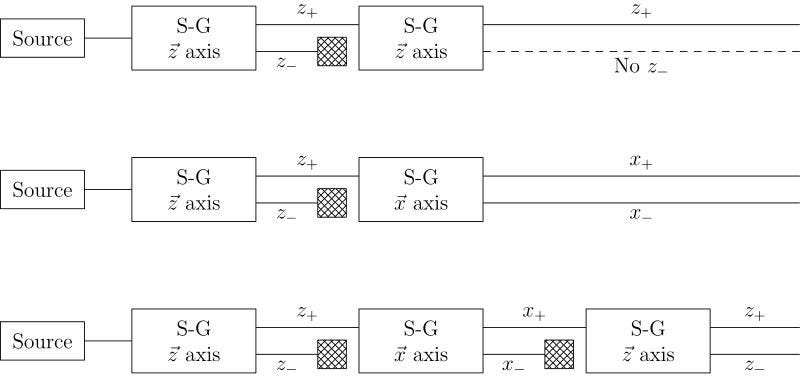Multiple successive Stern-Gerlach experiments, which split quantum particles along one axis according to their spins, will cause further magnetic splitting in directions perpendicular to the most recent one measured, but no additional splitting in the same direction. (FRANCESCO VERSACI OF WIKIMEDIA COMMONS)

But for quantum operators, order can matter tremendously. If you measure a quantum particle’s spin in the x-direction and then in the y-direction, the particle will have fundamentally different properties than if you measure it in the opposite order. This property ⁠ — known as non-commutivity ⁠ — requires complex, rather than real, mathematics (in particular, complex vector spaces) in order to explain it.

The fact that a complex number squared can give you a negative result led to a revolutionary mathematical solution to the Dirac equation, predicting the existence of “negative quantum states.” Dirac initially called these states “holes,” but shortly thereafter, physicists realized what was truly going on: this was the first theoretical prediction of antimatter, in the form of the anti-electron, or positron. Its experimental confirmation was one of the most important discoveries in the development of modern quantum physics.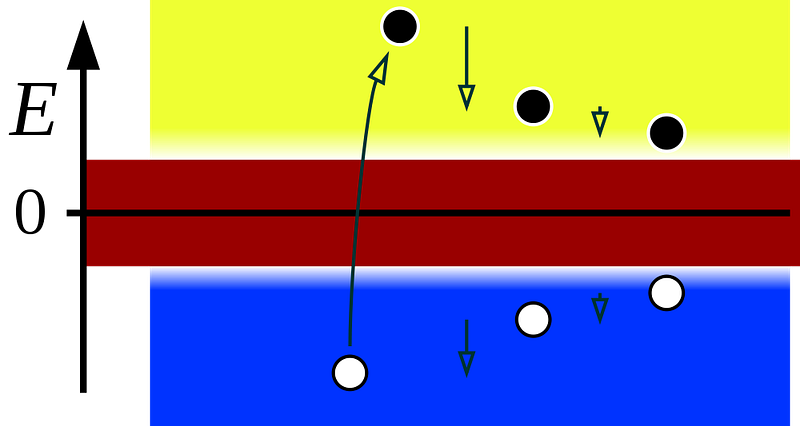The so-called ‘Dirac sea’ arose from solving the Dirac equation, based on a complex vector space, which yielded both positive and negative energy solutions. The negative solutions were soon identified with antimatter, and the positron (anti-electron) in particular, and opened up an entire new world for particle physics. (INCNIS MRSI / PUBLIC DOMAIN)

You might think, intuitively, that if you could find a more complicated, more general mathematical structure that extended the complex numbers ⁠ — the way the complex numbers extended the real ones ⁠ — you could find a new physical application. If you try to take the square root of a complex number, regardless of whether its real and imaginary parts are either positive or negative, you’ll always still get a complex number. This route won’t lead you to a richer mathematical structure.

But there’s an inherently non-commutative extension you could apply to the complex numbers: instead of letting = -1, you can define three independent entities, i, j, and k, where = = = -1, but where the combination i * j * k = -1 also. This fourfold set of factors, where instead of a real number (a) or a complex number (a + bi), you get what’s known as a quaternion: a + bi + cj + dk.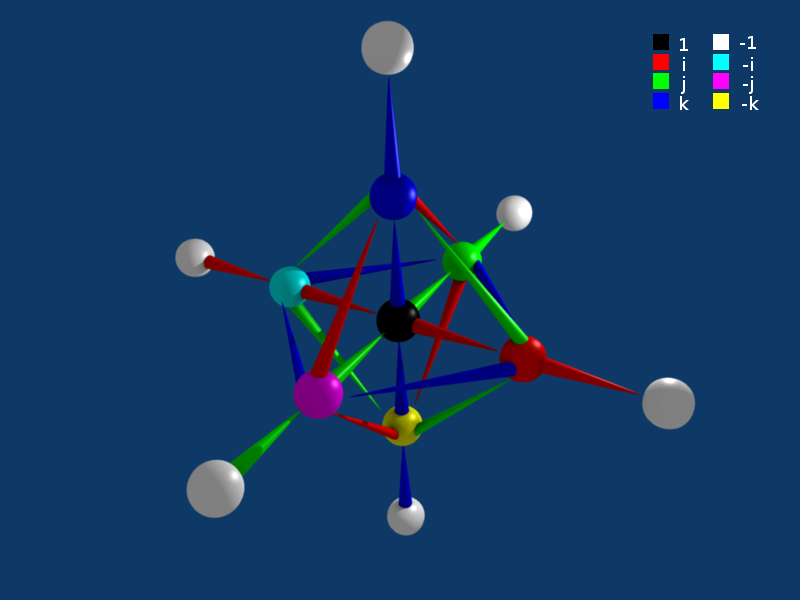This graph represents multiplication by the quaternion values i, j, and k, which are represented by red, green, and blue arrows, respectively. Note how they can transform between real, imaginary, and the other two fundamentally quaternion (j and k) numbers. (NIELMO / WIKIMEDIA COMMONS)

Quaternions are enormously useful in mathematics, but they are also relate to a large number of physical applications. Whereas a complex number represents points in a two-dimensional plane (with a real axis and an imaginary axis), a quaternion has enough dimensions and degrees-of-freedom in it to describe points in three-dimensional space.

The Lorentz transformations, which describe how lengths contract and time dilates as you move close to the speed of light, uses the quaternion group. The General Theory of Relativity can be related to the quaternions in modern algebra. The weak interactions involve quaternions, as do three-dimensional spatial rotations. Certain quantum phenomena are reversed if you rotate your system by 360 degrees, but come back to normal if you do it again and go 720 degrees.

Quaternions are fundamentally non-commutative, and explain why rotating a three-dimensional object about one axis and then another gives you a different final state than rotating that same object about the same two axes, but in the opposite order.The author’s last cellphone in the pre-smartphone era exemplifies how rotations in 3D space do not commute. At left, the top and bottom rows begin in the same configuration. At top, a 90 degree counterclockwise rotation in the plane of the photograph is followed by a 90 degree clockwise rotation around the vertical axis. At bottom, the same two rotations are performed but in the opposite order. This demonstrates the non-commutativity of rotations. (E. SIEGEL)

So, you might wonder, can you extend the quaternions even farther? Is there some other way to leverage mathematics where there’s another option available to open up an even richer structure?

The answer is yes, but it comes with a cost. The next step to a more complex mathematical structure is to go from the quaternions to the octonions, which have eight elements apiece, but it comes with a price. For quaternions, multiplication order matters, as Q1 * Q2 is not the same as Q2 * Q1, but the quaternions are still associative. If you have three quaternions (Q1, Q2, and Q3), then (Q1 * Q2) * Q3 = Q1 * (Q2 * Q3). But if you have three octonions, they’re both non-commutative and non-associative; multiplication order not only matters, but it matters in this fundamentally new way.Travel the Universe with astrophysicist Ethan Siegel. Subscribers will get the newsletter every Saturday. All aboard!

Whereas the mathematics of quaternions are related to a number of known physical theories, the mathematics of octonions is descriptive of operations that go beyond known physics, describing phenomena that appear in extensions such as Grand Unified Theories (GUTs) and string theory.Feynman diagrams (top) are based off of point particles and their interactions. Converting them into their string theory analogues (bottom) gives rise to surfaces which can have non-trivial curvature. In string theory, all particles are simply different vibrating modes of an underlying, more fundamental structure: strings. But do octonions, which have strong ties to string theory, actually have a role to play in our Universe? Or is it just math? (PHYS. TODAY 68, 11, 38 (2015))

Although applications of the octonions to physics are conjectural, there are many good reasons to be interested in these ideas. The octonions teach us, theoretically, how many spacetime dimensions you need to construct a supersymmetric quantum field theory. They are tied to the exceptional Lie groups which are used to construct GUTs and which play a role, through the E(8) group, in superstring theories.

The four classes of numbers we’ve just discussed — the real numbers, the complex numbers, the quaternions, and the octonions — are special in the mathematical field of abstract algebra. These four classes are the only algebras where you can always divide one number by any number other than zero and not get an undefined quantity, making them the only normed division algebras that exist.

If you try to extend the octonions to form a a 16-element algebra, you arrive at the sedenions, which obey their own non-commutative, non-associative multiplication rules, but fail if you try to incorporate division.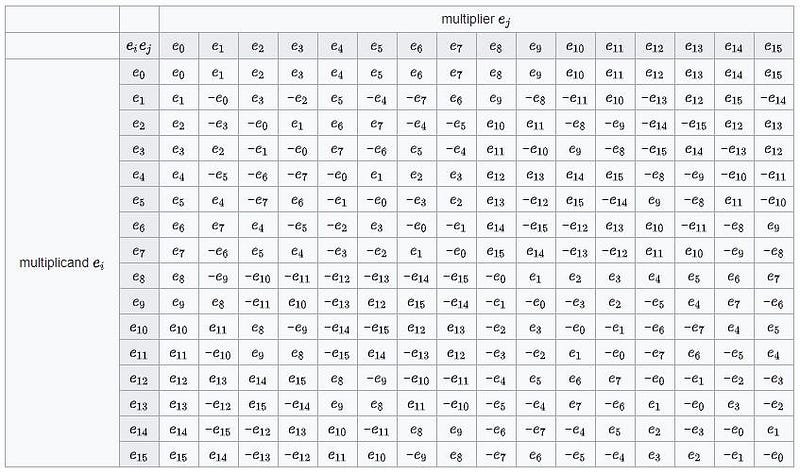The multiplication rules for the sedenions, the 16-element algebra that extends the 8-element octonions, works according to non-commutative, non-associative mathematical rules, which doesn’t pose a problem. But there is no normed division algebra for the sedenions, and that’s why we do not extend the octonions farther when looking for physical applications. (ENGLISH WIKIPEDIA)

The octonions themselves will never be “the answer” to how reality works, but they do provide a powerful, generalized mathematical structure that has its own unique properties. It includes real, complex, and quaternion mathematics, but also introduces fundamentally unique mathematical properties that can be applied to physics to make novel — but speculative and hitherto unsupported — predictions.

Octonions can give us an idea of which possibilities might be compelling to look at in terms of extensions to known physics and which ones might be less interesting, but there are no concrete observables predicted by the octonions themselves. Pierre Ramond, my former professor who taught me about octonions and Lie groups in physics, was fond of saying, “octonions are to physics what the Sirens were to Ulysses.” They definitely have an allure, but if you dive in, they may drag you to a hypnotic, inescapable doom.

Their mathematical structure holds an incredible richness, but nobody knows whether that richness means anything for our Universe or not.

Send in your Ask Ethan questions to startswithabang at gmail dot com!

Ethan Siegel is the author of Beyond the Galaxy and Treknology. You can pre-order his third book, currently in development: the Encyclopaedia Cosmologica.

Related
Lorem ipsum dolor sit amet, consectetur adipiscing elit. Nullam id tincidunt mi. Morbi malesuada nulla sit amet est hendrerit tincidunt. Etiam viverra, nisl id volutpat eleifend, est augue sodales orci, […]
Transfer of learning is a concept that should be top-of-mind when planning any learning and development program. Why? In a perfect world, the billions of dollars organizations collectively spend every […]
We thought the Big Bang started it all. Then we realized that something else came before, and it erased everything that existed prior.
There is more consensus on what heaven looks like than hell.
A group of prominent scientists shares how research has changed them.

Up Next
Big Think has launched a line of apparel and goods that celebrate the life and work of four geniuses.